# Process Capability

Process capability refers to the capability of a process to consistently make a product that meets a customer specified specification tolerance. Capability indices are used to predict the performance of a process by comparing the width of process variation to the width of the specified tolerance. It is used extensively in many industries and only has meaning if the process being studied is stable (in statistical control). Capability indices allow calculations for both short term (Cp and Cpk ) and/or long-term (Pp and Ppk) performance for a process whose output is measured using variable data at a specific opportunity for a defect.

The determination of process capability requires a predictable pattern of statistically stable behavior (most frequently a bell-shaped curve) where the chance causes of variation are compared to the engineering specifications. A capable process is a process whose spread on the bell-shaped curve is narrower than the tolerance range or specification limits. USL is the upper specification limit and LSL is the lower specification limit.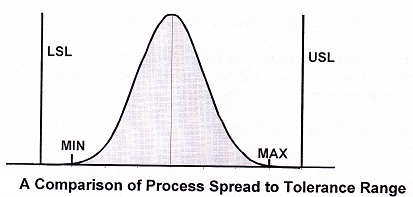It is often necessary to compare the process variation with the engineering or specification tolerances to judge the suitability of the process. Process capability analysis addresses this issue. A process capability study includes three steps:

• Planning for data collection
• Collecting data
• Plotting and analyzing the results

The objective of process quality control is to establish a state of control over the manufacturing process and then maintain that state of control through time. Actions that change or adjust the process are frequently the result of some form of capability study. When the natural process limits are compared with the specification range, any of the following possible courses of action may result:

• Do nothing. If the process limits fall well within the specification limits, no action may be required.
• Change the specifications. The specification limits may be unrealistic. In some cases, specifications may be set tighter than necessary. Discuss the situation with the final customer to see if the specifications may be relaxed or modified.
• Center the process. When the process spread is approximately the same as the specification spread, an adjustment to the centering of the process may bring the bulk of the product within specifications.
• Reduce variability. This is often the most difficult option to achieve. It may be possible to partition the variation (stream-to-stream, within piece, batch-to-batch, etc.) and work on the largest offender first. For a complicated process, an experimental design may be used to identify the leading source of variation.
• Accept the losses. In some cases, management must be content with a high loss rate (at least temporarily). Some centering and reduction in variation may be possible, but the principal emphasis is on handling the scrap and rework efficiently.

Other capability applications:

•  Providing a basis for setting up a variables control chart
•  Evaluating new equipment
• Reviewing tolerances based on the inherent variability of a process
• Assigning more capable equipment to tougher jobs
• Performing routine process performance audits
• Determining the effects of adjustments during processing

#### Identifying Characteristics

The identification of characteristics to be measured in a process capability study should meet the following requirements:

• The characteristic should be indicative of a key factor in the quality of the product or process.
• It should be possible to adjust the value of the characteristic.
• The operating conditions that affect the measured characteristic should be defined and controlled

If a part has ten different dimensions, process capability would not normally be performed for all of these dimensions. Selecting one, or possibly two, key dimensions provides a more manageable method of evaluating the process capability. For example in the case of a machined part, the overall length or the diameter of a hole might be the critical dimension. The characteristic selected may also be determined by the history of the part and the parameter that has been the most difficult to control or has created problems in the next higher level of  assembly. Customer purchase order requirements or industry standards may also determine the characteristics that are required to be measured. In the automotive industry, the Production Part Approval Process (PPAP)  states “An acceptable level of preliminary process capability must be determined prior to submission for all characteristics designated by the customer or supplier as safety, key, critical, or significant, that can be evaluated using variables (measured) data.” Chrysler, Ford and General Motors use symbols to designate safety and/or government regulated characteristics and important performance, fit, or appearance characteristics.

#### Identifying Specifications/Tolerances

The process specifications or tolerances, are determined either by customer requirements, industry standards, or the organization’s engineering department. The process capability study is used to demonstrate that the process is centered within the specification limits and that the process variation predicts the process is capable of producing parts within the tolerance requirements. When the process capability study indicates the process is not capable, the information is used to evaluate and improve the process in order to meet the tolerance requirements. There may be situations where the specifications or tolerances are set too tight in relation to the achievable process capability. In these circumstances, the specification must be reevaluated. If the specification cannot be opened, then the action plan is to perform 100% inspection of the process, unless inspection testing is destructive.

#### Developing Sampling Plans

The appropriate sampling plan for conducting process capability studies depends upon the purpose and whether there are customer or standards requirements for the study. Ford and General Motors specify that process capability studies for PPAP submissions be based on data taken from a signiﬁcant production run of a minimum
of 300 consecutive pieces.
If the process is currently running and is in control, control chart data may be used to calculate the process capability indices. If the process fits a normal distribution and is in statistical control, then the standard deviation can be estimated from:For new processes, for example for a project proposal, a pilot run may be used to estimate the process capability. The disadvantage of using a pilot run is that the estimated process variability is most likely less than the process variability expected from an ongoing process. Process capabilities conducted for the purpose of improving the process may be performed using a design of experiments (DOE) approach in which the optimum A values of the process variables which yield the lowest process variation is the  objective.

#### Verifying Stability and Normality

If only common causes of variation are present in a process, then the output of the process forms a distribution that is stable over time and is predictable. If special causes of variation are present, the process output is not stable over time.The Figure  depicts an unstable process with both process’ average and variation out-of-control. Note, the process may also be unstable if either the process average or variation is out-of-control. Common causes of variation refer to the many sources of variation within a process that has a stable and repeatable distribution over time. This is called a state of statistical control and the output of the process is predictable. Special causes refer to any factors causing variation that are not always acting on the process. If special causes of variation are present, the process distribution changes and the process output is not stable over time. When plotting a process on a control chart, lack of process stability can be shown by several types of patterns including: points outside the control limits, trends, points on one side of the center line, cycles, etc. The validity of the normality assumption may be tested using the chi square hypothesis test. To perform this test, the data is partitioned into data ranges. The number of data points in each range is then compared with the number predicted from a normal distribution. Using the hypothesis test with a selected confidence level, a conclusion can be made as to whether the data follows a normal distribution.
The chi square hypothesis test is:
Ho: The data follows a specified distribution
H1: The data does not follow a specified distribution
and is tested using the following test statistic: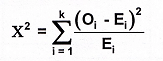Continuous data may be tested using the Kolmogorov-Smirnov goodness-of-fit test. It has the same hypothesis test as the chi square test, and the test statistic is givenWhere D is the test statistic and F is the theoretical cumulative distribution of the continuous distribution being tested. An attractive feature of this test is that the distribution of the test statistic does not depend on the underlying cumulative distribution function being tested. Limitations of this test are that it only applies to continuous distributions and that the distribution must be fully specified. The location, scale, and shape parameters must be specified and not estimated from the data. The Anderson-Darling test is a modification of the Kolmogorov-Smirnov test and gives more weight to the tails of the distribution.  If the data does not fit a normal distribution, the chi square hypothesis test may also be used to test the fit to other distributions such as the exponential or binomial distributions.

#### Capability index Failure Rates

There is a direct link between the calculated Cp (and Pp values) with the standard  normal (Z value) table.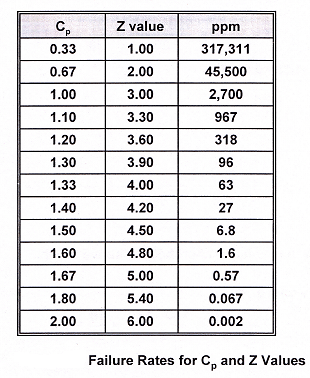A Cp of 1.0 is the loss suffered at a Z value of 3.0, ppm equals parts per million of nonconformance (or failure) when the process:

• is centered on Y
• Has a two-tailed specification
• Is normally distributed
• Has no significant shifts in average or dispersion

When the Cp, Cpk, Pp, and Ppk values are 1.0 or less, 2 values and the standard normal table can be used to determine failure rates. With the drive for increasingly dependable products, there is a need for failure rates in the Cp range of 1.5 to 2.0.

#### Process Capability Indices

To determine process capability, an estimation of sigma is necessary: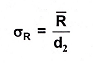(IR is an estimate of process capability sigma and comes from a control chart.
The capability index is defined as: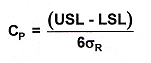As a rule of thumb:

• CR > 1.33 Capable
•  CR = 1.00 to 1.33 Capable with tight control
• CR < 1.00 incapable

The capability ratio is defined as:As a rule of thumb:

• CR < 0.75 Capable
• CR = 0.75 to 1.00 Capable with tight control
• CR > 1.00 incapable

Note, this rule of thumb logic is somewhat out of step with the six sigma assumption of a ±1.5 sigma shift. The above formulas only apply if the process is centered, stays centered within the speciﬁcations, and CR = CPR.

Cpk is the ratio giving the smallest answer between: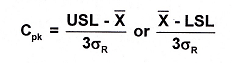For Example, For a process with  (Xbar)= 12, σR = 2 an USL =16 and LSL = 4, determine Cp and Cpk min: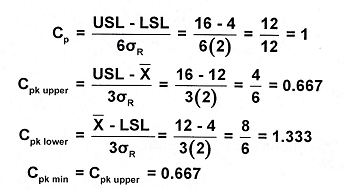##### Cpm index

The Cpm index is defined as:Where: USL = upper specification limit
LSL = lower specification limit
μ = process mean
T = target value
σ = process standard deviation
Cpm is based on the Taguchi index, which places more emphasis on process centering on the target.

For example for a process with μ = 12, σ = 2, T = 10, USL = 16 and LSL = 4, determine Cpm: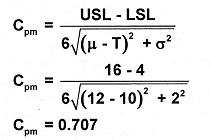##### Process Performance indices

To determine process performance, an estimation of sigma is necessary: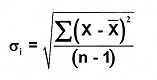σi is a measure of total data sigma and generally comes from a calculator or computer.
The performance index is defined as: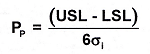The performance ratio is defined as:Ppk is the ratio giving the smallest answer between: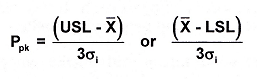### Short-Term and Long-Term Capability

Up to this point, process capability has been discussed in terms of stable processes, with assignable causes removed. In fact, the process average and spread are dependent upon the number of units measured or the duration over which the process is measured.
When a process capability is determined using one operator on one shift, with one piece of equipment, and a homogeneous supply of materials, the process variation is relatively small. As factors for time, multiple operators, various lots of material, environmental changes, etc. are added, each of these contributes to increasing the process variation. Control limits based on a short-term process evaluation are closer together than control limits based on the long-term process. A short run can be described with respect to time and a small run, where there is a small number of pieces produced. When a small amount of data is available, there is generally less variation than is found with a larger amount of data. Control limits based on the smaller number of samples will be narrower than they should be, and control charts will produce false out-of-control patterns. Smith suggests a modified X(bar) and R chart for short runs, running an initial 3 to 10 pieces without adjustment. A calculated value is compared with a critical value and either the process is adjusted or an initial number of subgroups is run. Inflated D4 and A2 values are used to establish control limits. Control limits are recalculated after additional groups are run. For small runs, with a limited amount of data,  X and MR chart can be used. The X represents individual data values, not an average, and the MR is the moving range, a measure of piece-to-piece variability. Process capability or Cpk values determined from either of these methods must be considered preliminary information. As the number of data points increases, the calculated process capability will approach the true capability. When comparing attribute with variable data, variable data generally provides more information about the process, for a given number of data points. Using variables data, a reasonable estimate of the process mean and variation can be made with 25 to 30 groups of five samples each. Whereas a comparable estimate using attribute data may require 25 groups of 50 samples each. Using variables data is preferable to using attribute data for estimating process capability.

#### Short-Term Capability Indices

The short-term capability indices Cp and Cpk are measures calculated using the short-term process standard deviation. Because the short-term process variation is used, these measures are free of subgroup drift in the data and take into account only the within subgroup variation. Cp is a ratio of the customer-specified tolerance to six standard deviations of the short-term process variation. Cp is calculated without regard to location of the data mean within the tolerance, so it gives an indication of what the process could perform to if the mean of the data was centered between the specification limits. Because of this assumption, Cp is sometimes referred to as the process potential. Cpk is a ratio of the distance between the process average and the closest specification limit, to three standard deviations of the short-term process variation. Because Cpk takes into account location of the data mean within the tolerance, it is a more realistic measure of the process capability. Cpk is sometimes referred to as the process performance.

#### Long-Term Capability Indices

The long-term capability indices Pp and Ppk are measures calculated using the long-term process standard deviation. Because the long-term process variation is used, these measures take into account subgroup drift in the data as well as the within subgroup variation. Pp is a ratio of the customer-specified tolerance to six standard deviations of the long-term process variation. Like Cp, Pp is calculated without regard to location of the data mean within the tolerance. Ppk is a ratio of the distance between the process average and the closest specification limit, to three standard deviations of the long-term process variation. Like Cpk, Ppk takes into account the location of the data mean within the tolerance. Because Ppk uses the long-term variation in the process and takes into account the process centering within the specified tolerance, it is a good indicator of the process performance the customer is seeing.

Because both Cp and Cpk are ratios of the tolerance width to the process variation, larger values of Cp and Cpk are better. The larger the Cp and Cpk, the wider the tolerance width relative to the process variation. The same is also true for Pp and Ppk. What determines a “good” value depends on the definition of “good.” A Cp of 1.33 is approximately equivalent to a short-term Z of 4. A Ppk of 1.33 is approximately equivalent to a long-term Z of 4. However, a Six Sigma process typically has a short term Z of 6 or a long-term Z of 4.5.Where σst = short-term pooled standard deviation.
And σlt = long-term standard deviation.

#### Manufacturing Example:

Suppose the diameter of a spark plug is a critical dimension that needs to conform to lower and upper customer specification limits of 0.480″ and 0.490″, respectively. Five randomly selected spark plugs are measured in every work shift. Each of the five samples on each work shift is called a subgroup. Subgroups have been collected for three months on a stable process. The average of all the data was 0.487″. The short-term standard deviation has been calculated and was determined to be 0.0013″. The long-term standard deviation was determined to be 0.019″.

To Calculate Cp and Cpk:
Cp = (0.490 – 0.480)/(6 x 0.0013) = 0.010/0.0078 = 1.28
Cpl = (0.487 – 0.480)/(3 x 0.0013) = 0.007/0.0039 = 1.79
Cpu = (0.490 – 0.487)/(3 x 0.0013) = 0.003/0.0039 = 0.77
Cpk = min (Cpl, Cpu)
Cpk = min (1.79, 0.77) = 0.77

To Calculate Pp and Ppk:
Pp = (0.490″ – 0.480″)/(6 x 0.019) = 0.0100/0.114 = 0.09
Ppl = (0.487 – 0.480)/(3 x 0.019) = 0.007/0.057 = 0.12
Ppu = (0.490 – 0.487)/(3 x 0.019) = 0.003/0.057 = 0.05
Ppk = min (Ppl, Ppu)
Ppk = min (0.12, 0.05) = 0.05

In this example, Cp is 1.28. Because Cp is the ratio of the specified tolerance to the process variation, a Cp value of 1.28 indicates that the process is capable of delivering product that meets the specified tolerance (if the process is centered). (A Cp greater than 1 indicates the process can deliver a product that meets the specifications at least 99.73% of the time.) Any improvements to the process to increase our value of 1.28 would require a reduction in the variability within our subgroups. Cp, however, is calculated without regard to the process centering within the specified tolerance. A centered process is rarely the case so a Cpk value must be calculated.
Cpk considers the location of the process data average. In this calculation, we are comparing the average of our process to the closest specification limit and dividing by three short-term standard deviations. In our example, Cpk is 0.77. In contrast to the Cp measurement, the Cpk measurement clearly shows that the process is incapable of producing product that meets the specified tolerance.
Any improvements to our process to increase our value of 0.77 would require a mean shift in the data towards the center of the tolerance and/or a reduction in the within subgroup variation. (Note: For centered processes, Cp and Cpk will be the same.) Our Pp is 0.09. Because Pp is the ratio of the specified tolerance to the process variation, a Pp value of 0.09 indicates that the process is incapable of delivering product that meets the specified tolerance. Any improvements to the process to increase our value of 0.09 would require a reduction in the variability within and/or between subgroups. Pp, however, is calculated without regard to  he process centering within the specified tolerance. A centered process is rarely the case so a Ppk value, which accounts for lack of process centering, will surely indicate poor capability for our process as well. (Note: For both Pp and Cp, we assume no drifting of the subgroup averages.) Ppk represents the actual long-term performance of the process and is the index that most likely represents what customers receive. In the example, Ppk is 0.05, confirming our Pp result of poor process performance. Any improvements to the process to increase our value of 0.05 would require a mean shift in the data towards the center of the tolerance and/or a reduction in the within subgroup and between subgroup variations.

Suppose a call center reports to its customers that it will resolve their issue within fifteen minutes. This fifteen minute time limit is the upper specification limit. It is desirable to resolve the issue as soon as possible; therefore, there is no lower specification limit. The call center operates twenty-four hours a day in eight-hour shifts. Six calls are randomly measured every shift and recorded for two months. An SPC chart shows the process is stable. The average of the data is 11.7 minutes, the short-term pooled standard deviation is 1.2 minutes, and the long-term standard deviation is 2.8 minutes.

To Calculate Cp and Cpk:

Cp = cannot be calculated as there is no LSL
Cpl = undefined
Cpu = (15 – 11.7)/(3 x 1.2) = 3.3/3.6 = 0.92
Cpk = min (Cpl, Cpu) = 0.92

To Calculate Pp and Ppk:
Pp = cannot be calculated as there is no LSL
Ppl = undefined
Ppu = (15 – 11.7)/(3 x 2.8) = 3.3/8.4 = 0.39
Ppk = min (Pplk, Ppu) = 0.39

In this example, we can only evaluate Cpk and Ppk as there is no lower limit. These numbers indicate that if we can eliminate between subgroup variation, we could achieve a process capability (Ppk) of 0.92, which is our current Cpk.

### Process Capability for Non-Normal Data

In the real world, data does not always fit a normal distribution, and when it does not, the standard capability indices does not give valid information because they are based on the normal distribution. The first step is a visual inspection of a histogram of the data. If all data values are well within the specification limits, the process would appear to be capable. One additional strategy is to make non-normal data resemble normal data by using a transformation. The question is which one to select for the specific situation. Unfortunately, the choice of the “best” transformation is generally not obvious.
The Box-Cox power transformations are given by: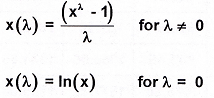Given data observations x1, x2,……. xn, select the power λ that maximizes the logarithm of the likelihood function: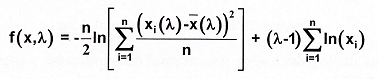Where the arithmetic mean of the transformed data is:Process capability indices and formulas described elsewhere in this Post are based on the assumption that the data are normally distributed. The validity of the normality assumption may be tested using the chi square hypothesis test. One approach to address the non-normal distribution is to make transformations to “normalize” the data. This may be done with statistical software that performs the Box-Cox transformation. As an alternative approach, when the data can be represented by a probability plot (i.e. a Weibull distribution) one should use the 0.135 and 99.865 percentiles to describe the spread of the data.

It is often necessary to identify non-normal data distributions and to transform them  into near normal distributions to determine process capabilities or failure rates Assume that a process capability study has been conducted. Some 30 data points from a non-normal distribution are shown in Table  below. An investigator can check the data for normality using techniques such as the dot plot, histogram, and normal probability plot.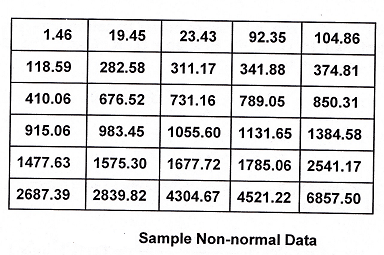A histogram displaying the above non-normal data indicates a distribution that is skewed to the right

.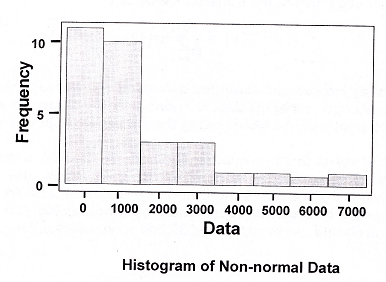A probability plot can also be used to display the non-normal data, The data points are clustered to the left with some extreme points to the right. Since this is a non- normal distribution, a traditional process capability index is meaningless.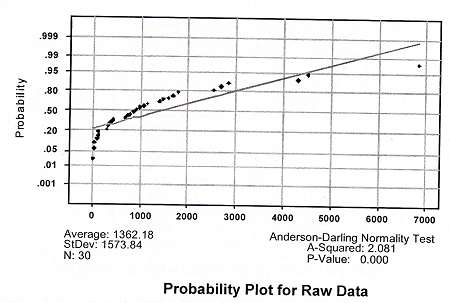If the investigator has some awareness of the history of the data, and knows it to follow a Poisson distribution, then a square root transformation is a possibility. The standard deviation is the square root of the mean. Some typical data transformations include:

• Log transformation (log x)
• Square root or power transformation (x y)
• Exponential (e y)
• Reciprocal (1/x)

In order to find the right transformation, some exploratory data analysis may be required. Among the useful power transformation techniques is the Box-Cox procedure. The applicable formula is:
y ’ =yλ
Where lambda, λ, is the power or parameter that must be determined to transform the data. For λ = 2, the data is squared. For λ = 0.5, a square root is needed.

One can also use Excel or Minitab to handle the data calculations and to draw the normal probability plot. With the use of Minitab, an investigator can let the Box-Cox tool automatically find a suitable power transform. in this example, a power transform of 0.337 is indicated. All 30 transformed data points from Table  above, using   y’ = y0.337, are shown in Table below.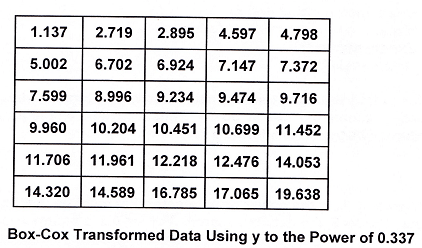A probability plot of the newly transformed data will show a near normal distribution.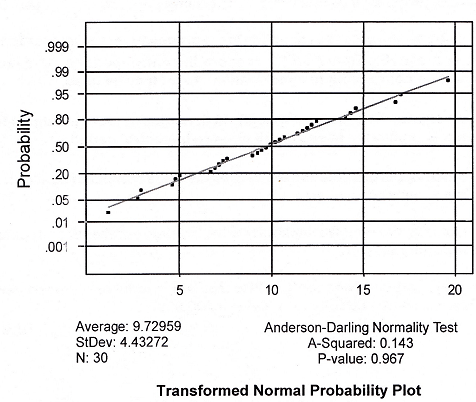Now, a process capability index can be determined forthe data. However, the investigator must remember to also transform the specifications. If the original specifications were 1 and 10,000, the new limits would be 1 and 22.28.

##### Process Capability for Attribute Data

The control chart represents the process capability, once special causes have been identified and removed from the process. For attribute charts, capability is deﬁned as the average proportion or rate of nonconforming product.

• for p charts, the process capability is the process average nonconforming, ̅p and is preferably based on 25 or more in-control periods. If desired, the proportion conforming to specification, 1-̅p may be used.
• for np charts, the process capability is the process average nonconforming, ̅p and is preferably based on 25 or more in-control periods.
• for c charts, the process capability is the process average nonconforming, ̅c in a sample of fixed size n.
• for u charts, the process capability is the process average nonconforming per reporting unit ̅u.

The average proportion of nonconformities may be reported on a defects per million opportunities scale by multiplying ̅p times 1,000,000.

Process Performance Metrics

• A defect is defined as something that does not conform to a known and accepted customer standard.
• A unit is the product, information, or service used or purchased by a customer.
• An opportunity for a defect is a measured characteristic on a unit that needs to conform to a customer standard (e.g., the ohms of an electrical resistor, the diameter of a pen, the time it takes to deliver a package, or the address field on a form).
• Defective is when the entire unit is deemed unacceptable because of the nonconformance of any one of the opportunities for a defect.
• Defects = D
• Opportunities (for a defect) = O
• Units = U
• Yield = Y

Defect Relationships

Defects per million opportunities (DPMO) helps to determine the capability of a process. DPMO allows for the calculation of capability at one or more opportunities and ultimately, if desired, for the entire organization.

Calculating DPMO depends on whether the data is variable or attribute, and if there is one or more than one opportunity for a defect. If there is:

• One opportunity with variable data, use the Z transform to determine the probability of observing a defect, then multiply by 1 million.
•  One opportunity with attribute data, calculate the percent defects, then multiple by 1 million.
• More than one opportunity with both variable and/or attribute data, use one of two methods to determine DPMO.
• To calculate DPO, sum the defects and sum the total opportunities for a defect, then divide the defects by the total opportunities and multiply by 1 million. For eg If there are eight defects and thirty total opportunities for a defect, then
DPMO = (8/30) x 1,000,000 = 266,667
• When using this method to evaluate multiple opportunity variable data, convert the calculated DPMO into defects and opportunities for each variable, then sum them to get total defects and opportunities. For eg. If one step in a process has a DPMO of 50,000 and another step has a DPMO of 100,000, there are 150,000 total defects for 2 million opportunities or 75,000 DPMO overall.
1. Total opportunities: T0 = TOP = U x O
2. Defects per unit: DPU = also = D/U= -ln (Y)
3. Defects per normalized unit: = -In (Ynorm)
4. Defects per unit opportunity= DPO = DPU/O=D/(Ux0)
5. Defects per million opportunities: DPMO = DPO x 106

for example  a matrix chart indicates the following information for 100 production units. Determine DPU. Assume that each unit in had 6 opportunities for a defect (i.e characteristics A, B, C, D, E, and F). Determine DPO and DPMO.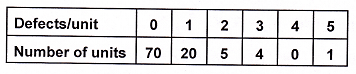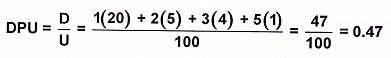One would expect to find an average of 0.47 defects per unit.
DPO = DPU/O=0.47/6 = 0.078333
DPMO = DPO x 106 = 78,333

#### Rolled Throughput Yield

Rolled Throughput Yield (RTY) is used to assess the true yield of a process that includes a hidden factory. A hidden factory adds no value to the customer and involves fixing things that weren’t done right the first time. RTY determines the probability of a product or service making it through a multistep process without being scrapped or ever reworked.

There are two methods to measure RTY:
Method 1 assesses defects per unit (dpu), when all that is known is the final number of units produced and the number of defects. Shown in the following diagram are six units, each containing five opportunities for a defect.Given that any one defect can cause a unit to be defective, it appears the yield of this process is 50%. This, however, is not the whole story. Assuming that defects are randomly distributed, the special form of the Poisson distribution formula
RTY = e-dpu
can be used to estimate the number of units with zero defects (i.e., the RTY). The previous figure showed eight defects over six units, resulting in 1.33 dpu. Entering this into our formula:
RTY = e-1.33
RTY = 0.264

According to this calculation, this process can expect an average of 26.4% defect-free units that have not been reworked (which is much different than the assumed 50%).

Method 2 determines throughput yield (Ytp), when the specific yields at each opportunity for a defect are known. If, on a unit, the yield at each opportunity for a defect is known (i.e., the five yields at each opportunity in the previous figure), then these yields can be multiplied together to determine the RTY. The yields at each opportunity for a defect are known as the throughput yields, which can be calculated as
Ytp = e-dpu
for that specific opportunity for a defect for attribute data, and
Ytp = 1- P(defect)
for variable data, where P(defect) is the probability of a defect based on the normal distribution. Shown in the following figure is one unit from the previous figure in which the associated Ytp’s at each opportunity were measured for many units.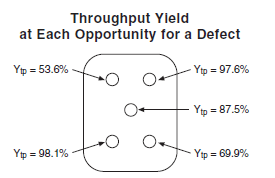Multiplying these yields together results in the RTY:
RTY = Ytp1 x Ytp2 x Ytp3 x Ytp4 x Ytp5
RTY = 0.536 x 0.976 x 0.875 x 0.981 x 0.699
RTY = 0.314
According to this calculation, an average of 31.4% defect free
units that have not been reworked can be expected.

###### Yield Relationships

Note, the Poisson equation is normally used to model defect occurrences. If there is a historic defect per unit (DPU) level for a process, the probability that an item contains X flaws (PX) is described mathematically by the equation: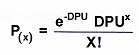Where:    X is an integer greater or equal to 0
DPU is greater than 0
Note that 0! (zero factorial) = 1 by definition.

If one is interested in the probability of having a defect free unit (as most of us are), then X = 0 in the Poisson formula and the math is simplified:
P(0) = e-dpu
Therefore, the following common yield formulas follow:
Yield or first pass yield: Y = FPY = e-dpu .
Defects per unit: DPU = – ln (Y) (In means natural logarithm)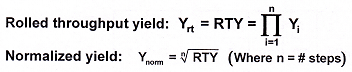Total defects per unit: TDPU = -ln (Ynorm)

For example the yield for  a process has a DPU of 0.47 is
Y = e-dpu = e-0.47 = 0.625 = 62.5%
For example the DPU for a process with a first pass yield of 0.625 is
DPU = -ln(Y) = -ln 0.625 = 0.47
Example: A process consists of 4 sequential steps: 1, 2, 3, and 4. The yield of each step is as follows: Y1 = 99%, Y, =98%, Y3 = 97%, Y4 = 96%. Determine the rolled throughput yield and the total defects per unit.
Yrt  = (0.99)(0.98)(0.97)(0.96) = 0.90345 = 90.345%
TDPU = -ln(RTY) = —ln 0.90345 = 0.1015

Rolled throughput yield is defined as the cumulative calculation of yield or defects through multiple process steps. The determination of the rolled throughput yield (RTY) can help a team focus on serious improvements.

• Calculate the yield for each step and the resulting RTY
• The RTY for a process will be the baseline metric
• Revisit the project scope
• Significant yield differences can suggest improvement opportunities

#### Sigma Relationships

Probability of a defect = P(d)
P(d)=1-Y or 1 – FPY
also P(d) = 1 – Yrt (for a series of operations)

P(d) can be looked up in a Z table (using the table in reverse to determine Z).

The Z value determined  is called Z long-term or Z equivalent.
Z short-term is defined as: Zst = Zlt + 1.5 shift

For example  the Z short-term for   Z long-term = 1.645,  is
Zst = Zlt +1.5 =1.645 +1.5 = 3.145

Schmidt and Launsby  report that the 6 sigma quality level (with the 1.5 sigma shift) can be approximated by:
6 Sigma Quality Level = 0.8406 + SQRT(29.37 – 2.221 x In (ppm))

Example: If a process were producing 80 defectives/million, what would be the 6 sigma quality level?
6σ = 0.8406 + SQRT(29.37 – 2.221 x In (80))
6σ = 0.8406 + SQRT(29.37 – 2.221 (4.3820))
6σ= 0.8406 + 4.4314 = 5.272 (about 5.3)